# Transient in a coupled circuit

Homework Helper
Gold Member

## Homework Statement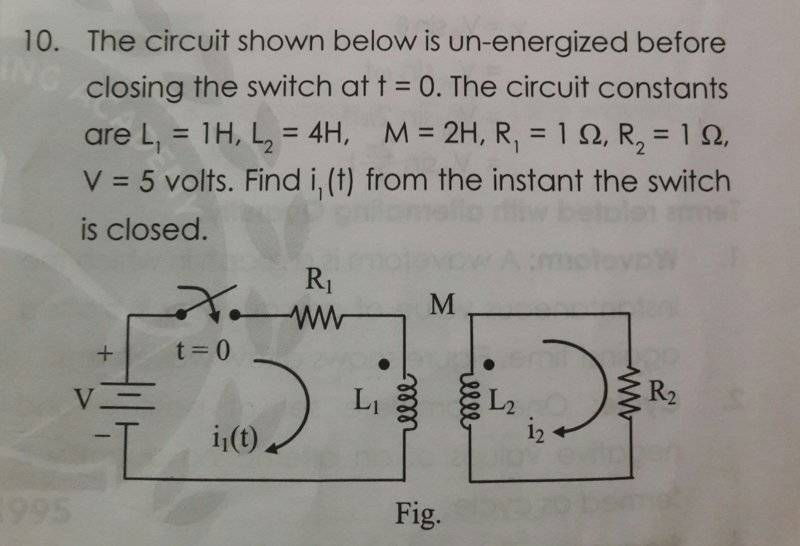Kirchhoff's laws

## The Attempt at a Solution

Applying KVL on primary,
di1/dt+i1=5+2di2/dt.....1)

Similarly for secondary,
2di1/dt= 4di2/dt + i2.....2)

After solving these equations using Laplace Transform, I got
I1= 5-e-0.2t A and
I2 = 2e-0.2t-2e-0.25t.

I have verified these answers using initial and final conditions and I believe they are correct.

However, at t=0, the energy in the system comes out to be 8J (E= 0.5L1i12+0.5L2I22-Mi1i2, since there is magnetic opposition).

How is this possible? How can the energy change instantaneously here?
For E to be zero at t=0, i2 at t=0 should be 2A. But here, i2|t=0= 0A.

What am I missing here?

#### Attachments

Grinkle
Gold Member
I1 (0) looks to be 4A per your solution to I1, which does not seem right. I1(0) and I2(0) both need to be zero, yes?

gneill
Mentor
After solving these equations using Laplace Transform, I got
I1= 5-e-0.2t A and
I2 = 2e-0.2t-2e-0.25t.
Your I1 looks good, but I2 is suspicious. How does a second time constant arise?
However, at t=0, the energy in the system comes out to be 8J (E= 0.5L1i12+0.5L2I22-Mi1i2, since there is magnetic opposition).

How is this possible? How can the energy change instantaneously here?
For E to be zero at t=0, i2 at t=0 should be 2A. But here, i2|t=0= 0A.
Blame it on the mutual inductance. Since ##\frac{dI}{dt}## will be nonzero at ##t = 0## for both loops, there will be induced currents regardless of the "rule of thumb" behavior that we assume for isolated inductors.

•cnh1995
Homework Helper
Gold Member
How does a second time constant arise?
I will check the math again. But I2 should be zero at t=0 and at t=∞. I believe a single time constant can't make that happen.

Also, in the KVL equation, while taking LT of di1/dt, I wrote sI1(s)-i1(0) and put i1(0)=0. How come it is not that same in the final expression for i1?

Last edited:
Homework Helper
Gold Member
I will check the math again. But I2 should be zero at t=0 and at t=∞. I believe a single time constant can't make that happen.

Also, in the KVL equation, while taking LT of di1/dt, I wrote sI1(s)-i1(0) and put i1(0)=0. How come it is not same in the final expression of i1?
Plus, di/dt at t=0 in a normal series R-L circuit is E/L (non-zero). I didn't understand your point about di/dt being non-zero here.

gneill
Mentor
The fact that the currents will be changing means that there will be changing flux though the loops each caused by the changing current in the other loop. Then, regardless of the usual "I = 0 at t = 0 for an inductor", Faraday's law says that a changing flux in a loop will induce an EMF.

When I do these sorts of problems I usually re-draw the circuit and write in the Laplace Domain values for the circuit components. Then write the equations by inspection. So for your circuit,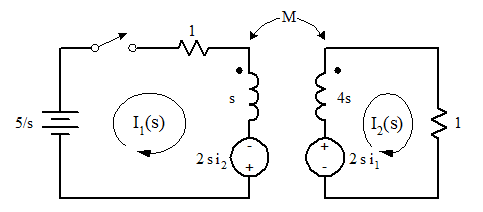hence: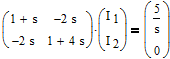By the way, I've confirmed the results of the above analysis using LTSpice to simulate the circuit: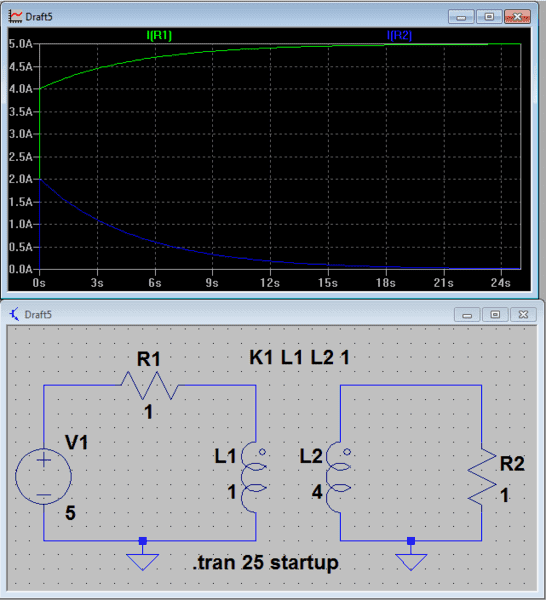As you can see, ##i_1## does indeed start off at 4 amps, and ##i_2## at 2 amps. Goes against intuition, but the math bears it out.

#### Attachments

•cnh1995 and Grinkle
Homework Helper
Gold Member
I also ran a simulation on my phone and it is giving me the same result.Goes against intuition, but the math bears it out.
Well, I believe I2 must start off at 2A as per the energy equation in the OP.

I am getting the exact same equations in the s-domain as you are, but my answer for i2 has two time constants.

Also,
Also, in the KVL equation, while taking LT of di1/dt, I wrote sI1(s)-i1(0) and put i1(0)=0. How come it is not that same in the final expression for i1?
Why is this happening?

Homework Helper
Gold Member
As you can see, i1i1i_1 does indeed start off at 4 amps, and i2i2i_2 at 2 amps. Goes against intuition, but the math bears it out.
On the secondary side, as per the KVL equations, mutually induced emf is the "source" and L2 and R2 behave as the load.
At t=0, this mutually induced emf Mdi1/dt equals 0.4V.
How come the secondary current is 2A? That would require at least 2V in the secondary.

This circuit is crazy!

gneill
Mentor
I am getting the exact same equations in the s-domain as you are, but my answer for i2 has two time constants.

Let me cheat and use Mathcad to do all the work: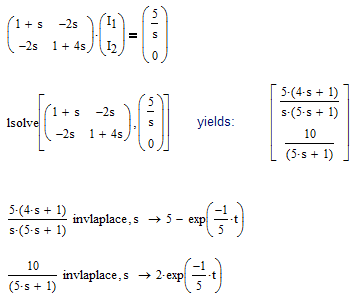Also, in the KVL equation, while taking LT of di1/dt, I wrote sI1(s)-i1(0) and put i1(0)=0. How come it is not that same in the final expression for i1?
Again, Faraday's law says that the induced EMF will be spread out all along the loop. While we model mutual inductance as a simple source in series with the inductor, it's really not. The current induced by the EMF is not truly the ##I_1## of the diagram.

Edit:
What I meant to say is, the 5 V source is not the only source of EMF driving current ##I_1##. And the induction based contribution is driven by Faraday.

#### Attachments

•cnh1995
Homework Helper
Gold Member
Yes, when you solve both the s-domain equations simultaneously, you get i2=2A and that doesn't violate the physics of circuits!Perhaps my thinking below is incorrect because the Laplace equations have something to do with discontinuity of the current function at t=0.
On the secondary side, as per the KVL equations, mutually induced emf is the "source" and L2 and R2 behave as the load.
At t=0, this mutually induced emf Mdi1/dt equals 0.4V.
How come the secondary current is 2A? That would require at least 2V in the secondary.

This circuit is crazy!

I found the expression for i1(t), then found the expression for Mdi1/dt and then took the LT of the equation
Mdi1/dt= i2+4di2/dt.
If you solve this, you get
I2 = 2e-0.2t-2e-0.25t.

Why is this method incorrect?

gneill
Mentor
I found the expression for i1(t), then found the expression for Mdi1/dt and then took the LT of the equation
Mdi1/dt= i2+4di2/dt.
If you solve this, you get
I2 = 2e-0.2t-2e-0.25t.
Why is this method incorrect?
I'm not sure, but perhaps because you lose the fact that there is an initial "DC" current of 5 amps when you differentiate in the time domain? If I do the differentiation in the Laplace domain (simply by multiplying ##I_1(s)## by ##s##), then I have

##2 \frac{5 (4 s + 1)}{(5 s + 1)} = I_2(s) + 4 s I_2(s)##

and

##I_2(s) = \frac{10}{5 s + 1} ##

•cnh1995
Homework Helper
Gold Member
I'm not sure, but perhaps because you lose the fact that there is an initial "DC" current of 5 amps when you differentiate in the time domain? If I do the differentiation in the Laplace domain (simply by multiplying ##I_1(s)## by ##s##), then I have

##2 \frac{5 (4 s + 1)}{(5 s + 1)} = I_2(s) + 4 s I_2(s)##

and

##I_2(s) = \frac{10}{5 s + 1} ##
I agree, that must be it!I had removed the 5A dc simply by taking its derivative in the time domain.

•gneill
rude man
Homework Helper
Gold Member
It may be noted that it's fortunate that the coefficient of coupling is unity.
If for example M=1 instead of 2 we would get I1(s) = (4s+1)/[s(3s2+5s+1)] with pretty tortuous inversion math. Ditto for I2.

•cnh1995